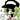# The Little Schemer 知识点整理（九）：……周而复始……

2019 年 01 月 24 日

## 自定义函数

### (looking a lat)

``````(define looking
(lambda (a lat)
(keep-looking a (pick 1 lat) lat)))``````
``````(define keep-looking
(lambda (a sorn lat)
(cond
((number? sorn)
(keep-looking a (pick sorn lat) lat))
(else (eq? sorn a)))))``````

sorn 代表 symbol or number。

### (eternity x)

``````(define eternity
(lambda (x)
(eternity x)))``````

### (shift pair)

``````(define shift
(lambda (pair)
(build (first (first pair))
(build (second (first pair))
(second pair)))))``````

### (align pora)

`align``keep-looking` 一样，在第二个条件中，`(shift pora)` 改变了 `align` 的参数，它不一定是 pora 的一部分，无法保证能够接近最终目标。其实这是违反了第七诫。

``````(define align
(lambda (pora)
(cond
((atom? pora) pora)
((a-pair? (first pora))
(align (shift pora)))
(else (build (first pora)
(align (second pora)))))))``````

### (length* pora)

``````(define length*
(lambda (pora)
(cond
((atom? pora) 1)
(else
(plus (length* (first pora))
(length* (second pora)))))))``````

### (shuffle pora)

``````(define shuffle
(lambda (pora)
(cond
((atom? pora) pora)
((a-pair? (first pora))
(shuffle (revpair pora)))
(else (build (first pora)
(shuffle (second pora)))))))``````

### (A n m)

``````(define A
(lambda (n m)
(cond
((zero? m) (A (sub1 n) 1))
(else (A (sub1 n)
(A n (sub1 m)))))))``````

## 停机问题

``````(define will-stop?
(lambda (f)
...))``````

1. length

`(length (quote ()))` 是 0，这时 `will-stop? length` 为 #t。

2. eternity

`(eternity (quote ()))` 不会返回值，根本停不下来，这时 `will-stop? length` 显然是 #f。

3. last-try

定义 `last-try`

``````(define last-try
(lambda (x)
(and (will-stop? last-try)
(eternity x))))``````

`(last-try (quote ()))` 的值也就是 `(and (will-stop? last-try) (eternity (quote ())))` 的值。考虑以下两种情况：

• `(will-stop? last-try)` 是 #f，表示 `last-try` 停不下来，则：

1. `(and (will-stop? last-try) (eternity (quote ())))` = #f；
2. `(last-try (quote ()))` = #f，表示 `last-try` 能够停下来。嗯？矛盾。
• `(will-stop? last-try)` 是 #t，表示 `last-try` 能够停下来，则：

1. `(and (will-stop? last-try) (eternity (quote ())))`，后面的 `eternity` 停不下来，整体也停不下来啊；
2. 于是 `(last-try (quote ()))` 也停不下来。嗯？？？也矛盾了。

## Y 组合子

EOFTwinkle 的博客